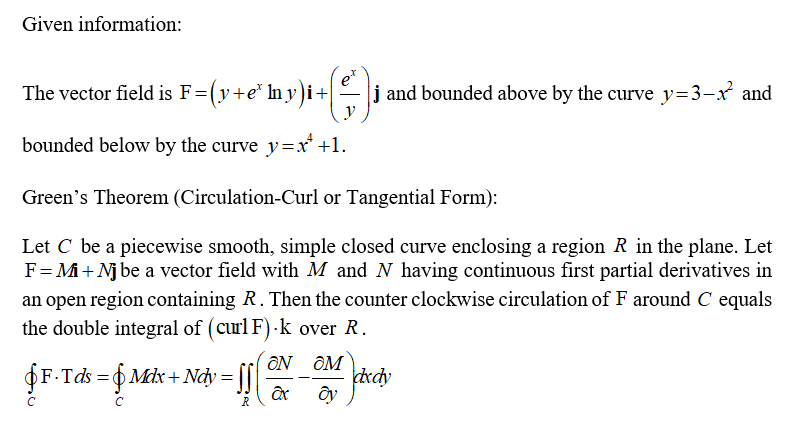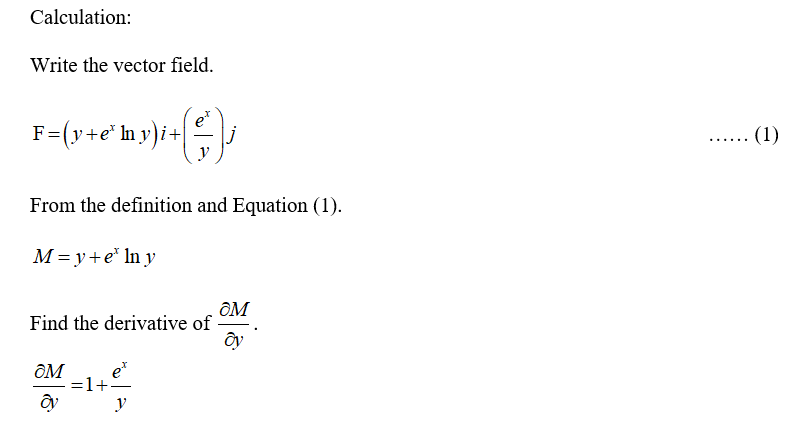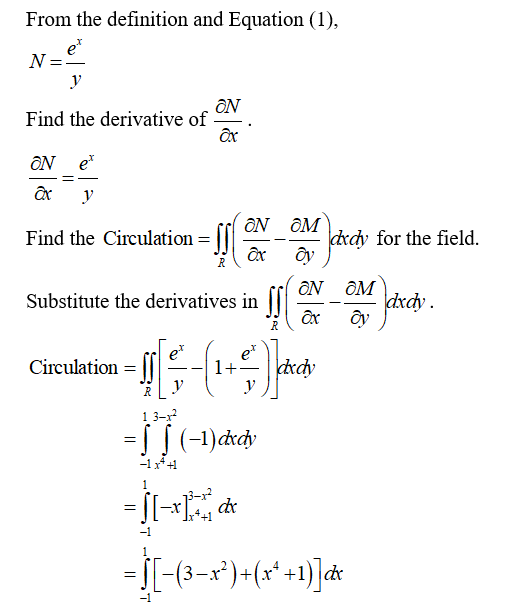# Find the counterclockwise circulation of F = (y + ex ln y)i + (ex/y)j around the boundary of the region that is bounded above by the curve y = 3 - x2 and below by the curve y = x4 +1

Question
1 views

Find the counterclockwise circulation of F = (y + ex ln y)i + (ex/y)j around the boundary of the region that is bounded above by the curve y = 3 - x2 and below by the curve y = x4 +1

check_circle

Step 1Step 2Step 3...

### Want to see the full answer?

See Solution

#### Want to see this answer and more?

Solutions are written by subject experts who are available 24/7. Questions are typically answered within 1 hour.*

See Solution
*Response times may vary by subject and question.
Tagged in